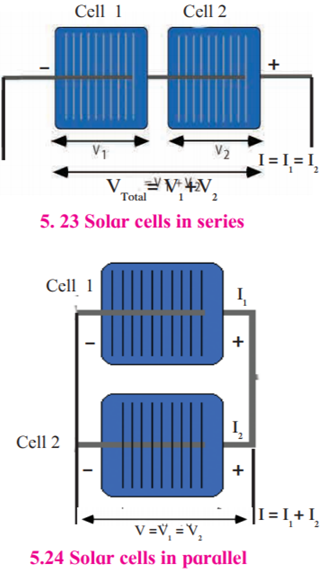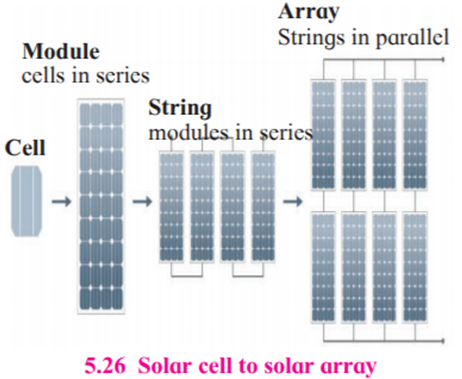SSC BOARD PAPERS IMPORTANT TOPICS COVERED FOR BOARD EXAM 2024

### Answer the following questions. a. How can we get the required amount of energy by connecting solar panels?

Question 11.

a. How can we get the required amount of energy by connecting solar panels?[T1]

We can get the required amount of energy by connecting solar photovoltaic cells which convert the solar energy directly into electrical energy. This method is called solar photovoltaic effect.

We get DC supply from the solar panels. Thus, equipment that require DC supply can be directly used whereas for those appliances which demand AC supply, the DC solar power is converted into AC power using an inverter.

These solar panels are semiconductors, usually made out of silicon.

A silicon solar cell of dimension 1 square cm generates current of about 30mA and potential difference of about 0.5V.Series connection of solar cells: The potential difference obtained from this combination is addition of the potential differences of individual solar cells. However, the current generated from this combination is equal to the current from an individual cell.

Parallel connection of solar cells: If two solar cells are connected in parallel, the current generated from this combination is the summation of the currents from an individual solar cells. However, the potential difference obtained from this combination is the same as the potential difference obtained from individual cell.

Solar panels are connected in series and parallel to generate required DC current and potential difference.

Many solar panels connected in series form solar strings and solar strings connected in parallel form a solar array. Thus, required electrical power is obtained and is used in applications which need marginal power to power station of MW capacity.